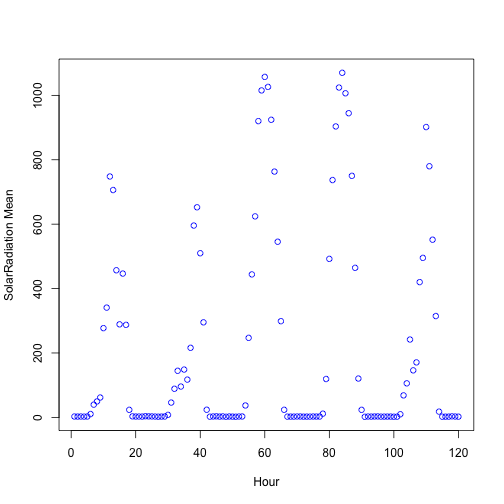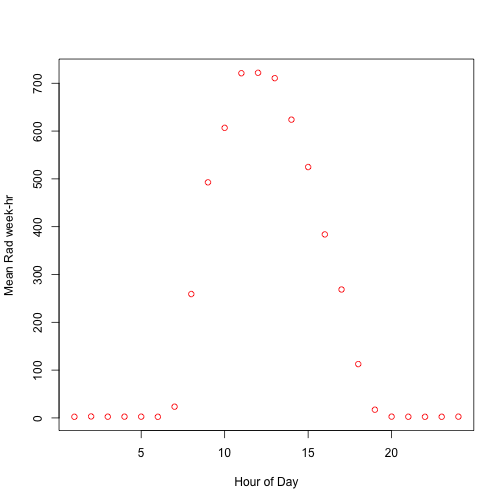# Data Cleanup with Solar Radiation Data Set

## 2017/12/09

#### Purpose

Before you can run a statistical analysis, you may have to clean data. This post is all about using some techniques to clean data. This is not necessarily the best way, or even the correct way to do this; however, it is mean to generate your own ideas for cleaning data in the future.

#### Data Location

The data comes from the Kaggle site here. It contains over 32,000 observations of Solar Radiation data.

#### The Goal

The goal is to use historical data from multiple input parameters to correctly predict levels of solar radiation. We can get a glimpse of the data and data types using the head function. The following list shows the 11 column names and corresponding data type from the raw data file.

• Parameters

• UNIXTime (int) - Number of seconds since Jan 1, 1970

• Data (fctr) - MM/DD/YYY 12:00:00 AM

• Time (fctr) - Hawaii time (HH:MM:SS)

• Temperature (int) - degrees Fahrenheit

• Pressure (dbl) - unknown

• Humidity (int) - percent

• WindDireciton.Degrees. (dbl) degrees

• Speed (dbl) - miles/hour

• TimeSunRise (fctr) - Hawaii time (HH:MM:SS)

• TimeSunSet (fctr) - Hawaii time (HH:MM:SS)

#### Code and Explanations

First, we want to set up the enviornment to make things a big easier.

In this post, I will descirbe the sections that do not seem obvious only. Read the comments within the code to get some insight as to what is going on.

##### Start / Stop Clock

StartTime is simply a variable to keep track of how long it takes to run this code. More for curiosity than anything else. You will see how this get’s calculated at the end.

#####################################################################
#Set Up of Enviornment
#####################################################################
StartTime = Sys.time()

##### Create Logger File

It is sometimes important to log big events. I created this just to get some practice with logging. This chuck of code will create a log file called “SOLAR_LOGGER” and append today’s date. This way, everything you log will be captured in today’s log file only. Tomorrow, a new log file will be created if you run the code. This can help keep track of changes.

#Set up a logger file system
LoggerFile = 'SOLAR_LOGGER'

#Create a log file using LoggerFile + Append today's Date
fileDate = paste(LoggerFile, Sys.Date(), sep = " ")
fileDate = paste(fileDate, ".txt")

#Create Daily Log File
if (!file.exists(fileDate)){
write("Solar Logger File Created ",
fileDate)
} else {
if (existsFunction("function.logger")){
function.logger("File overwrite denied!",2)
} else {
warning("function.logger() Not Executed Yet")
}
}


Note that the above code only created the actual file.

##### Logger Function

Now, we will create the logger function. Feel free to to change the typeError messages to suit your needs.

#Logger Function
function.logger = function(logInput = "No Input Defined", typeError = 1) {
lineAppend = paste(Sys.time() , logInput, sep = " ")

if (typeError == 1){
message("See Logger")
}
if(typeError == 2){
warning("See Logger")
}
if (typeError == 3){
stop("EXECUTION STOPPED: See Logger")
}

write(lineAppend, file = fileDate, append = TRUE)
}

function.logger("LOGGER FILE")

<code>## See Logger
</code>


The logger file has been created. You should see a LOGGERFILE text file in the working directory now.

##### Import Data

Next we import the data, if it exists. It’s available on the kaggle site an later I will upload it to this post for direct download as well. The name of the file is “SolarPrediction.csv” and it has a header.

#####################################################################
#####################################################################

SolarCSV = "SolarPrediction.csv"
if (file.exists(SolarCSV)){
} else {
function.logger("File missing. stop() called.", 3)
}

##### Column Names

Now that the Solar data frame is created, click on the enviornment variable to view the header and contents. Notice the “Data” column should really read “Date.” Let’s change that.

#Rename "Data" field to 'Date' for readability
names(Solar)[names(Solar) == 'Data'] = 'Date'
function.logger("Renamed Data column to Date")

attach(Solar)

##### Libraries

Install proper libraries.

#Libraries
if(!require(chron)){
install.packages("chron")
library(chron)
function.logger("Installed chron package.", 1)
}

if(!require(tseries)){ install.packages("tseries")
library(tseries)
function.logger("Installed tseries package.", 1)
}

if(!require(data.table)){ install.packages("data.table")
library(data.table)
function.logger("Installed data.table package.", 1)
}


Drop redundant data, convert time into chron time objects and create new variables Hour, Minute, and DayLength

#####################################################################
#Clean Data
#####################################################################

drops <- c("UNIXTime") #List of items to drop from Data.Frame
Solar = Solar[ , !(names(Solar) %in% drops)]
function.logger("Removed UNIXTime from Data.")

#Convert date/times to date/time objects
Solar$TimeSunRise = chron(times = as.character(TimeSunRise)) Solar$TimeSunSet = chron(times = as.character(TimeSunSet))
Solar$Hour = as.numeric(format(strptime(Time,"%H:%M:%S"),'%H')) #Show just the Hour Solar$Minute = format(strptime(Time, "%H:%M:%S"), '%M') #Show just the Minute
Solar$Date = as.character(Solar$Date)
Solar$Date = substr(Solar$Date,1, nchar(Solar$Date)-12) Solar$Date = chron(date = Solar$Date, #Strip time off - invalid data ######FLAG format = "m/d/y") #Time component not valid, removed #Calculate Length of Day Solar$DayLength = Solar$TimeSunSet - Solar$TimeSunRise


I never used this chuck but it may be useful.

#Assignments for Averages Data
HumH=(aggregate( Humidity ~ Hour, Solar, mean ))
PresH=(aggregate( Pressure ~ Hour, Solar, mean ))
WinH=(aggregate( WindDirection.Degrees. ~ Hour, Solar, mean ))
SpeedH=(aggregate( Speed ~ Hour, Solar, mean ))

##### Wind Direction

Having wind directions of say, 359 degrees an 2 degrees are vastly different numerically; however, they are essentially the same thing as saying “North.” The next chuck simply converts wind direction numerics into factors of N, E, W, and S.

Even though I converted these, I did not use them in analysis yet. How should I average directions over an hour’s period? Or over a week? Based on frequeny of occurance? I have’t had time to figure that out yet, perhaps you can find a good use for it.

#Convert Wind Direction into Factors N, E, W, S based on values
function.WindDirToFactors = function(degVect){
result = "ERROR"
if (degVect >= 315 || degVect < 45) { result = "N" }
if (degVect >= 45 && degVect < 135) { result = "E" }
if (degVect >= 135 && degVect < 225){ result = "S" }
if (degVect >= 225 && degVect < 315){ result = "W" }

if (result == "Error"){
function.logger("Wind Direction out of Range", 1)
}

return(result)
}

##### Week Number

I wanted to look at weekly averages so this function helps convert dates into the actual week number of the year, 1-52.

#Convert Date to the Numbered Week of the Year
function.weekofyear <- function(dt) {
as.numeric(format(as.Date(dt), "%W"))
}

#Calculate Week Number and Assign Wind Directions to Data
WeekNum = rep(0, length(Solar$Date)) WindDirection.Factors = rep(0, length(Solar$WindDirection.Degrees.)) #Place Holder
for (i in 1:length(WindDirection.Factors)){
WindDirection.Factors[i] = function.WindDirToFactors(Solar$WindDirection.Degrees.[i]) WeekNum[i] = function.weekofyear(Solar$Date[i])
}

#Create New Column "WeekNum" and attach
Solar$WeekNumber = WeekNum function.logger("Added WeekNumber column.", 1) WindDirection.Factors = as.factor((WindDirection.Factors)) Solar$WndDirFact = WindDirection.Factors #add wind dir factors to original data.frame

#Reattach Solar for use of new columns without scoping
detach(Solar)
attach(Solar)

##### Grouping Data

I highly recommend using Data Tables rather than Data Frames for this next chunk of code. Data Tables allow SQL type queires direclty in the column or row areas of the brackets. It automatically groups them by grp_cols and applies whatever function you want to the parameters.

In the first case, we group by mean values of the actual DATE and HOUR. This way, we can see, by the hour what the averages are. The other groupings are similar.

Finally, I order the data by date.

#####################################################################
#Aggregate Data
#####################################################################

#Using data.table for aggregtion features
Solar.dt = data.table(Solar)
Solar.dt.byweek = data.table(Solar)
Solar.dt.byWkHr = data.table(Solar)

#Group DATE with HOUR
grp_cols = c(names(Solar) , names(Solar)) #Columns to Group By
PresMean = mean(Pressure),
TempMean = mean(Temperature),
HumMean = mean(Humidity),
SpeedMean = mean(Speed),
WeekNumMean = mean(WeekNumber)),
by = grp_cols]

#Group by WEEK
grp_cols = c(names(Solar)) #Columns to Group By
PresMean = mean(Pressure),
TempMean = mean(Temperature),
HumMean = mean(Humidity),
SpeedMean = mean(Speed)),
by = grp_cols]

#Group by WEEK-HOUR
grp_cols = c(names(Solar), names(Solar)) #Columns to Group By
PresMean = mean(Pressure),
TempMean = mean(Temperature),
HumMean = mean(Humidity),
SpeedMean = mean(Speed)),
by = grp_cols]

#####################################################################
#Order Data
#####################################################################

#Order Data
Solar = Solar[order(Date),]
Solar.dt = Solar.dt[order(Solar.dt$WeekNumMean),] Solar.dt.byweek = Solar.dt.byweek[order(Solar.dt.byweek$WeekNumber),]
Solar.dt.byWkHr = Solar.dt.byWkHr[order(Solar.dt.byWkHr$WeekNumber, Solar.dt.byWkHr$Hour),]

##### Output Files

I chose to create output files for various ways the data was manipulated. Mainly because I am going to work as a team for the analysis portion of this possibly. I want to be able to send just the data to teammates and not let them worry about cleaning.

#####################################################################
#Output .csv Files for Statistics
#####################################################################
write.csv(Solar, file = "Solar1.csv", row.names = FALSE)
write.csv(Solar.dt, file = "Solar2.csv", row.names = FALSE)
write.csv(Solar.dt.byweek, file = "Solar3.csv", row.names = FALSE)
write.csv(Solar.dt.byWkHr, file = "Solar4.csv", row.names = FALSE)

function.logger("Created Solar1.csv - Cleaned Version of Original", 1)
function.logger("Created Solar2.csv - Average Values by Hour", 1)
function.logger("Created Solar3.csv - Average Values by Week", 1)
function.logger("Created Solar4.csv - Average Values by Week-Hour", 1)

##### Runtime Stats

This is the part of the code that can give us an idea of how long the cleansing process took.

#####################################################################
#Runtime Statistics - Goes at END OF FILE
#####################################################################
detach(Solar)
EndTime = Sys.time()
TotalTime = EndTime - StartTime

RunTime = paste("Total Run Time = ", round(TotalTime,4), "seconds.")
function.logger(RunTime)

plot(Solar.dt$RadMean[1:120], ylab = "SolarRadiation Mean", xlab = "Hour", col = "blue")plot(Solar.dt.byWkHr$RadMean[1:24], col = "red", ylab = "Mean Rad week-hr", xlab = "Hour of Day")#####################################################################

##### Next Steps

To be honest, this is rather crude and I am sure there is a lot of wasted steps and more elegant ways of doing this. As I learn the R language, I hope to improve naturally. Sometimes you just have to get things done though.

There are some hours with 0 observations, we may want to impute or discard that data. Just note that their are still some problems with this data. I will expand this post if I end up cleaning it up a bit more.